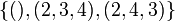# A3 in A5

(diff) ← Older revision | Latest revision (diff) | Newer revision → (diff)
This article is about a particular subgroup in a group, up to equivalence of subgroups (i.e., an isomorphism of groups that induces the corresponding isomorphism of subgroups). The subgroup is (up to isomorphism) cyclic group:Z3 and the group is (up to isomorphism) alternating group:A5 (see subgroup structure of alternating group:A5).
VIEW: Group-subgroup pairs with the same subgroup part | Group-subgroup pairs with the same group part | All pages on particular subgroups in groups

Let$G$ be the alternating group:A5, i.e., the alternating group (the group of even permutations) on the set$\{ 1,2,3,4,5 \}$.$G$ has order$5!/2 = 60$.

Consider the subgroup:$H = H_{\{1,2,3 \}} = \{ (), (1,2,3), (1,3,2) \}$$H$ has a total of 10 conjugate subgroups (including$H$ itself) and the subgroups are parametrized by subsets of size 3 in$\{1,2,3,4,5 \}$ describing the support of the 3-cycles. The complementary subset of size two is fixed point-wise by that conjugate subgroup:

Subset of size 3 Complementary subset of size 2 fixed point-wise by the subgroup Conjugate of$H$
1,2,3 4,5$\! \{ (), (1,2,3), (1,3,2) \}$
1,2,4 3,5$\! \{ (), (1,2,4), (1,4,2) \}$
1,2,5 3,4$\! \{ (), (1,2,5), (1,5,2) \}$
1,3,4 2,5$\! \{ (), (1,3,4), (1,4,3) \}$
1,3,5 2,4$\! \{ (), (1,3,5), (1,5,3) \}$
1,4,5 2,3$\! \{ (), (1,4,5), (1,5,4) \}$
2,3,4 1,5$\! \{ (), (2,3,4), (2,4,3) \}$
2,3,5 1,4$\! \{ (), (2,3,5), (2,5,3) \}$
2,4,5 1,3$\! \{ (), (2,4,5), (2,5,4) \}$
3,4,5 1,2$\! \{ (), (3,4,5), (3,5,4) \}$

Each of these subgroups is isomorphic to cyclic group:Z3.

## Arithmetic functions

Function Value Explanation
order of the whole group 60$5!/2 = 120/2 = 60$. See alternating group:A5.
[[order of a group|order of the subgroup 3
index of the subgroup 20 Follows from Lagrange's theorem
size of conjugacy class of subgroups = index of normalizer 10
number of conjugacy classes in automorphism class 1

## Effect of subgroup operators

In the table below, we provide values specific to$H$.

Function Value as subgroup (descriptive) Value as subgroup (link) Value as group
normalizer$\langle (1,2,3), (1,2)(4,5) \rangle$ twisted S3 in A5 symmetric group:S3
centralizer the subgroup itself current page cyclic group:Z3
normal core trivial subgroup -- trivial group
normal closure the whole group -- alternating group:A5
characteristic core trivial subgroup -- trivial group
characteristic closure the whole group -- alternating group:A5

## Conjugacy class-defining functions

Conjugacy class-defining function What it means in general Why it takes this value
Sylow subgroup for the prime$p = 3$ A$p$-Sylow subgroup is a subgroup whose order is a power of$p$ and index is relatively prime to$p$. Sylow subgroups exist and Sylow implies order-conjugate, i.e., all$p$-Sylow subgroups are conjugate to each other. The order of this subgroup is 3, which is the largest power of 3 dividing the order of the group.

## Related subgroups

### Intermediate subgroups

Value of intermediate subgroup (descriptive) Isomorphism class of intermediate subgroup Number of conjugacy classes of intermediate subgroup fixing subgroup and whole group Subgroup in intermediate subgroup Intermediate subgroup in whole group$\langle (1,2,3), (1,2)(4,5) \rangle$ symmetric group:S3 1 A3 in S3 twisted S3 in A5$\langle (1,2,3), (1,2)(3,4) \rangle$ alternating group:A4 1 A3 in A4 A4 in A5

## Description in terms of alternative interpretations of the whole group

Description of$G$ Corresponding description of$H$
special linear group of degree two over field:F4 Group of diagonal matrices of determinant 1.
projective special linear group of degree two over field:F5 If we think of the field$\mathbb{F}_{25}$ as a two-dimensional vector space over field:F5, its multiplicative group embeds in$GL(2,5)$. The intersection with$SL(2,5)$ corresponds to the elements whose sixth power is the identity. Projecting down to$PSL(2,5)$ gives a cyclic group of order three, which is precisely this subgroup.

## Subgroup properties

### Sylow and corollaries

The subgroup is a 3-Sylow subgroup, so many properties follow as a corollary of that.

Property Meaning Why being Sylow implies the property
order-conjugate subgroup conjugate to any subgroup of the same order Sylow implies order-conjugate
isomorph-conjugate subgroup conjugate to any subgroup isomorphic to it (via order-conjugate)
automorph-conjugate subgroup conjugate to any subgroup automorphic to it (via isomorph-conjugate)
intermediately isomorph-conjugate subgroup conjugate to any subgroup isomorphic to it inside any intermediate subgroup Sylow implies intermediately isomorph-conjugate
intermediately automorph-conjugate subgroup automorph-conjugate in every intermediate subgroup (via intermediately isomorph-conjugate)
pronormal subgroup conjugate to any conjugate subgroup in their join Sylow implies pronormal
weakly pronormal subgroup (via pronormal)
paranormal subgroup (via pronormal)
polynormal subgroup (via pronormal)

### Normality-related properties

Property Meaning Satisfied? Explanation Comment
normal subgroup equals all its conjugate subgroups No (see above for other conjugate subgroups)
2-subnormal subgroup normal subgroup in its normal closure No
subnormal subgroup series from subgroup to whole group, each normal in next No
contranormal subgroup normal closure is whole group Yes
self-normalizing subgroup equals its normalizer in the whole group No normalizer is twisted S3 in A5
self-centralizing subgroup contains its centralizer in the whole group Yes